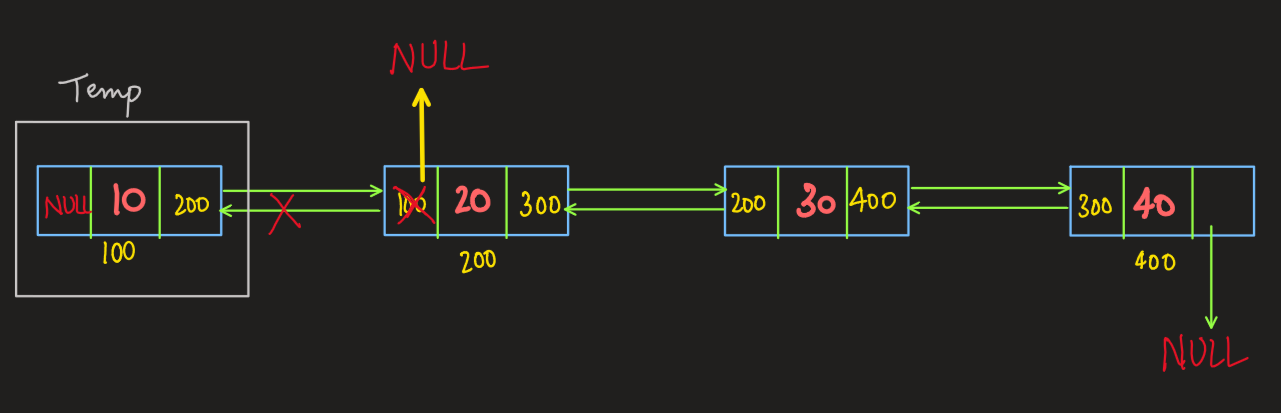# Delete Head of a Doubly Linked List

Problem Statement: Delete Head of a Doubly Linked List

Given a doubly linked list, your task is to delete the head node and return the modified list.

Examples:

```Example 1:
Input: 10 20 30
Output: 20 30

Note: The above input represents a doubly linked list.

Explanation: Since the input represents a doubly linked list, “10” is the head. After deletion, the linked list becomes “20 30”

Example 2:
Input: 10
Output: null or NULL

Explanation: Since “10” is the head of the doubly linked list which after deletion makes the linked list empty, hence we print “NULL” or “null”.

Example 3:
Input: NULL or null
Output: NULL or null
Explanation: Since the doubly linked list is empty, we print “NULL” or “null”```

DisclaimerDon’t jump directly to the solution, try it out yourself first.

## Solution:

### Intuition:

In this problem, we have to delete the head of the doubly linked list. So let’s imagine a doubly linked list. When we think of the head of a doubly linked list. It would have data, previous and next as its attributes. The previous of the head would point to NULL since the head is the first element of the DLL.

There are certain edge cases in this problem

The first edge caseDLL is empty.

We return NULL if DLL is empty because we have no head to delete.

Second edge caseDLL consists of a single node.

We delete the single node since it is the head and return NULL.

### Approach:

To delete the head, we need to detach it from the linked list which can be done in a very simple way.

1. We store our head into a temporary node.
2. We make the neighboring node as the new head
3. We change the previous pointer of the new head to point to NULL.
4. We delete the temporary node.

The following diagram may help in understanding the above approachCode:

## C++ Code

``````#include<iostream>

using namespace std;

struct node { // creating a node
int data;
node * previous;
node * next;
node(int d) {
data = d;
previous = NULL;
next = NULL;
}
};
if (head == NULL) { // checks if the DLL is empty [edge case:1]
return NULL;
}
if (head -> next == NULL) { // checks if the DLL has a single
element [edge case:2]
return NULL;
}
else {
node * temp = head; //stores the old head
head = head -> next; //makes the neighboring node as head
head -> previous = NULL; // changes the previous pointer for the new head
delete temp; //deletes the old head
}
}
void print_DLL(node * head) {
node * current = head;
while (current != NULL) {
cout << current -> data << " ";
current = current -> next;
}
cout<<endl;
}
int main() {
//creating nodes for the DLL
node * head = new node(10);
node * node1 = new node(20);
node * node2 = new node(30);
node * node3 = new node(40);

//constructing the DLL
head -> next = node1;
node1 -> previous = head;
node1 -> next = node2;
node2 -> previous = node1;
node2 -> next = node3;
node3 -> previous = node2;
cout<<"Original Doubly Linked List"<<endl;
cout<<"After deleting the head node"<<endl;
print_DLL(head); // this is a utility function
return 0;
}
``````

Output:

Original Doubly Linked List
10 20 30 40
After deleting the head node
20 30 40

Time Complexity: O(1)

Space Complexity: O(1)

## Java Code

``````import java.util.*;
import java.io.*;
import java.lang.*;

class node {
int data;
node previous;
node next;
node(int d) {
data = d;
previous = null;
next = null;
}
}

class DLL {

public static void main(String args[]) {
// creating nodes for the DLL
node head = new node(10);
node node1 = new node(20);
node node2 = new node(30);
node node3 = new node(40);

// constructing the DLL
node1.next = node2;
node2.previous = node1;
node2.next = node3;
node3.previous = node2;
System.out.println("Original Doubly Linked List");
System.out.println("After deleting the head node");
print_DLL(head); // utility function

}

if (head == null) return null; //checks if the DLL is empty
if (head.next == null) { //checks if the DLL contains a single element
return null;
} else {
head.previous = null; //changes the previous pointer for new head
}
}

public static void print_DLL(node head) {
node current = head;
while (current != null) {
System.out.print(current.data + " ");
current = current.next;
}
System.out.println("");
}
}
``````

Output:

Original Doubly Linked List
10 20 30 40
After deleting the head node
20 30 40

Time Complexity: O(1)

Space Complexity: O(1)

Special thanks to Yash Mishra for contributing to this article on takeUforward. If you also wish to share your knowledge with the takeUforward fam, please check out this article